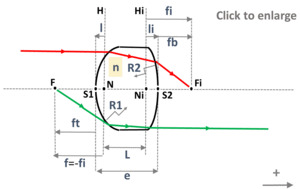and orgeometrical optics
focal lengths and principal planes

# thick lens

Caution
Fill in all input cells.
Choose all parameters unit.
n can be either a value or the reference of a glass from the catalog. If a value is directly entered, the wavelength will not be considered.
e is positive.
Raddii and x are algebric or infinite. Enter "infinite" for a plane surface or an object at infinity.
Positive direction is according the horizontal arrow in the bottom right of the scheme.

InputValueunit
n
R1
R2
e
OutputValueUnit
fito be calculated
lto be calculated
lito be calculated
Lto be calculated
ftto be calculated
fbto be calculatedR1 : radius of curvature of the first surface R2 : radius of curvature of the second surface l : distance from the vertex of the first surface to the primary principal plane li : distance from the vertex of the second surface to the secondary principal plane L : distance from the primary principal plane to the secondary principal plane

Note
This calculation page can not detect all unrealistic inputs.